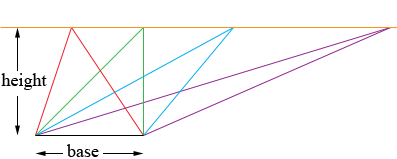SEARCH HOMEMath Central Quandaries & QueriesQuestion from Zack, a student: How would i calculate the height of a triangle if the base is 10cm and the area is 50cm²? If possible how would i calculate the length around the the triangle.Hi Zack,

The area of a triangle is given by the expression

area = 1/2 × base × height

You know the area and the base measurements so if you substitute these values into the expression above you can solve for the height.

The distance around a triangle is called its perimeter. In my diagram below I have 4 triangles, all with the same height and base and yet the perimeters are quite different.PennyMath Central is supported by the University of Regina and The Pacific Institute for the Mathematical Sciences.